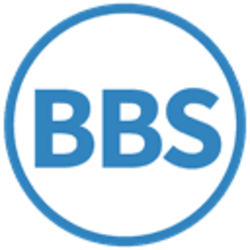# Quinary

Use Wikipedia with dynamical search help in all languages ...

Wikipedia - How to create a pageQuinary /ˈkwnəri/ (base-5 or pental) is a numeral system with five as the base. A possible origination of a quinary system is that there are five digits on either hand.

In the quinary place system, five numerals, from 0 to 4, are used to represent any real number. According to this method, five is written as 10, twenty-five is written as 100 and sixty is written as 220.

As five is a prime number, only the reciprocals of the powers of five terminate, although its location between two highly composite numbers (4 and 6) guarantees that many recurring fractions have relatively short periods.

Today, the main usage of base 5 is as a biquinary system, which is decimal using five as a sub-base. Another example of a sub-base system, is sexagesimal, base 60, which used 10 as a sub-base.

Each quinary digit can hold log25 (approx. 2.32) bits of information.

 × 1 2 3 4 10 11 12 13 14 20 1 1 2 3 4 10 11 12 13 14 20 2 2 4 11 13 20 22 24 31 33 40 3 3 11 14 22 30 33 41 44 102 110 4 4 13 22 31 40 44 103 112 121 130 10 10 20 30 40 100 110 120 130 140 200 11 11 22 33 44 110 121 132 143 204 220 12 12 24 41 103 120 132 144 211 223 240 13 13 31 44 112 130 143 211 224 242 310 14 14 33 102 121 140 204 223 242 311 330 20 20 40 110 130 200 220 240 310 330 400
Quinary Binary Decimal 0 1 2 3 4 5 6 7 8 9 0 1 2 3 4 10 11 12 13 14 20 21 22 0 1 10 11 100 101 110 111 1000 1001 1010 1011 1100 23 24 30 31 32 33 34 40 41 42 43 44 100 1101 1110 1111 10000 10001 10010 10011 10100 10101 10110 10111 11000 11001
 Decimal (periodic part) Quinary (periodic part) Binary (periodic part) 1/2 = 0.5 1/2 = 0.2 1/10 = 0.1 1/3 = 0.3 1/3 = 0.13 1/11 = 0.01 1/4 = 0.25 1/4 = 0.1 1/100 = 0.01 1/5 = 0.2 1/10 = 0.1 1/101 = 0.0011 1/6 = 0.16 1/11 = 0.04 1/110 = 0.010 1/7 = 0.142857 1/12 = 0.032412 1/111 = 0.001 1/8 = 0.125 1/13 = 0.03 1/1000 = 0.001 1/9 = 0.1 1/14 = 0.023421 1/1001 = 0.000111 1/10 = 0.1 1/20 = 0.02 1/1010 = 0.00011 1/11 = 0.09 1/21 = 0.02114 1/1011 = 0.0001011101 1/12 = 0.083 1/22 = 0.02 1/1100 = 0.0001 1/13 = 0.076923 1/23 = 0.0143 1/1101 = 0.000100111011 1/14 = 0.0714285 1/24 = 0.013431 1/1110 = 0.0001 1/15 = 0.06 1/30 = 0.013 1/1111 = 0.0001 1/16 = 0.0625 1/31 = 0.0124 1/10000 = 0.0001 1/17 = 0.0588235294117647 1/32 = 0.0121340243231042 1/10001 = 0.00001111 1/18 = 0.05 1/33 = 0.011433 1/10010 = 0.0000111 1/19 = 0.052631578947368421 1/34 = 0.011242141 1/10011 = 0.000011010111100101 1/20 = 0.05 1/40 = 0.01 1/10100 = 0.000011 1/21 = 0.047619 1/41 = 0.010434 1/10101 = 0.000011 1/22 = 0.045 1/42 = 0.01032 1/10110 = 0.00001011101 1/23 = 0.0434782608695652173913 1/43 = 0.0102041332143424031123 1/10111 = 0.00001011001 1/24 = 0.0416 1/44 = 0.01 1/11000 = 0.00001 1/25 = 0.04 1/100 = 0.01 1/11001 = 0.00001010001111010111

## Usage

Many languages use quinary number systems, including Gumatj, Nunggubuyu, Kuurn Kopan Noot, Luiseño and Saraveca. Gumatj is a true "5–25" language, in which 25 is the higher group of 5. The Gumatj numerals are shown below:

Number Base 5 Numeral
1 1 wanggany
2 2 marrma
3 3 lurrkun
4 4 dambumiriw
5 10 wanggany rulu
10 20 marrma rulu
15 30 lurrkun rulu
20 40 dambumiriw rulu
25 100 dambumirri rulu
50 200 marrma dambumirri rulu
75 300 lurrkun dambumirri rulu
100 400 dambumiriw dambumirri rulu
125 1000 dambumirri dambumirri rulu
625 10000 dambumirri dambumirri dambumirri rulu

## Biquinary

A decimal system with 2 and 5 as a sub-bases is called biquinary, and is found in Wolof and Khmer. Roman numerals are an early biquinary system. The numbers 1, 5, 10, and 50 are written as I, V, X, and L respectively. Seven is VII and seventy is LXX. The full list is:

 I V X L C D M 1 5 10 50 100 500 1000

Note that these are not positional number systems. In theory a number such as 73 could be written as IIIXXL without ambiguity as well as LXXIII and it is still not possible to extend it beyond thousands. There is also no sign for zero. But with the introduction of inversions such as IV and IX, it was necessary to keep the order from most to least significant.

Many versions of the abacus, such as the suanpan and soroban, use a biquinary system to simulate a decimal system for ease of calculation. Urnfield culture numerals and some tally mark systems are also biquinary. Units of currencies are commonly partially or wholly biquinary.

Bi-quinary coded decimal is a variant of biquinary that was used on a number of early computers including Colossus and the IBM 650 to represent decimal numbers.

A vigesimal system with 4 and 5 as a sub-bases is found in Nahuatl.[citation needed][dubious ]

## Calculators and programming languages

Few calculators support calculations in the quinary system, except for some Sharp models (including some of the EL-500W and EL-500X series, where it is named the pental system) since about 2005, as well as the open-source scientific calculator WP 34S.

Python's `int()` function supports conversion of numeral systems from any base to base 10. Thus, the quinary number 101 is evaluated using `int('101',5)` as 26.This page is funded by cryptomining